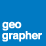# Help with dynamic label python code

1458
3
07-14-2016 01:36 PMNew Contributor II

Hello friend. I am pretty basic with Python and have a question about writing out a label.

I have an attribute table with multiple fields with floating point acreages in the fields. Not all of the fields necessarily have to contain a value; most of them are <Null>.

I am trying to build a label expression for a data driven map book that looks at the fields, and if it it has a value, add it to the label with leading text, then look at the next field. So far, I have using the IF statement and once the statement is true, it stops and does not search for the other true values.

Is there any If-and statement that I can use?

My code:

Function FindLabel ( [BLM] , [BR] , [Private] , [SITLA] )

if ( ( [BLM] ) > 0) then

FindLabel = "BLM: " + [BLM] + " acres"

elseif  ( ( [BR] ) > 0) then

FindLabel = "BR: " + [BR] + " acres"

elseif  ( ( [Private] ) > 0) then

FindLabel = "Private: " + [Private] + " acres"

elseif  ( ( [SITLA] ) > 0) then

FindLabel = "SITLA: " + [SITLA] + " acres"

end if

End Function

Assuming that there were acreages in each of the fields except [BR], I would want the label to read

BLM: 44.59 acres

Private: 89.01 acres

SITLA: 1.89 acres

Thanks for any help!

Tags (3)
1 Solution

Accepted SolutionsbyMVP Regular Contributor

Here's a rewrite of your FindLabel function.  It uses Blake's suggestions; there are a number of ways to do the same thing in Python.

```def FindLabel ([BLM], [BR], [Private], [SITLA]):
label_str = ""
if [BLM] : label_str += "BLM: {} acres\n".format([BLM])
if [BR] : label_str += "BR: {} acres\n".format([BR])
if [Private] : label_str += "Private: {} acres\n".format([Private])
if [SITLA] : label_str += "SITLA: {} acres\n".format([SITLA])
return label_str.strip()```
3 RepliesbyMVP Regular Contributor

If you are wanting to print the possible combinations, you wouldn't use an elseif.  Try something like this (but adapted for FindLabel):

```BLM = 5.5
BR = None
PVT =  6.5
SITLA = 7.5
label_str = ""

if BLM : label_str = "BLM: " + str(BLM) + " acres\n"
if BR : label_str = label_str + "BR: " + str(BR) + " acres\n"
if PVT : label_str = label_str + "PVT: " + str(PVT) + " acres\n"
if SITLA : label_str = label_str + "SITLA: " + str(SITLA) + " acres\n"

find_label = label_str
print find_label```byMVP Regular Contributor

If you use str.format() it will automatically make the non-string values text instead of having to do it manually. You can also use += to add to an existing value.

`label_str += "BR: {} acres\n".format(BR)`byMVP Regular Contributor

Here's a rewrite of your FindLabel function.  It uses Blake's suggestions; there are a number of ways to do the same thing in Python.

```def FindLabel ([BLM], [BR], [Private], [SITLA]):
label_str = ""
if [BLM] : label_str += "BLM: {} acres\n".format([BLM])
if [BR] : label_str += "BR: {} acres\n".format([BR])
if [Private] : label_str += "Private: {} acres\n".format([Private])
if [SITLA] : label_str += "SITLA: {} acres\n".format([SITLA])
return label_str.strip()```